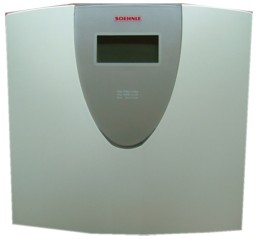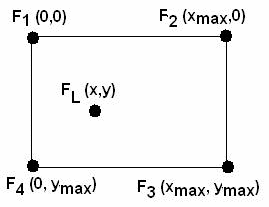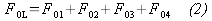We are closed until COVID-19 restrictions are lifted - there is no shipment and limited support during this period

# Convert an electronic personal weighing scale to measure center of pressure (Posturography)Warning: This application note illustrates a typical strain gage application and is not for actual medical use.

One use of Center of Pressure measurement is in the study of standing posture - Posturography. By measuring the position of the Center of Pressure at regular intervals, a plot called a spaghetti diagram can be generated. By calculating the mean and standard deviation of the center of pressure, an indication of sway is derived1.

In the paper Context Acquisition Based on Load Sensing2, an algorithm is given to measure the center of pressure using four load cells. If the total force exerted at x,y by the load is FL and assuming static forces, the sum of all 4 load cells F1, F2, F3, and F4 is equal to FL as given by eq 1Similarly, the no load force F0L is the sum of the forces on each load cell F01, F02, F03, and F04 - eq 2. The position of the load can be calculated by eq 3 and 4 using the load and no load forces.An electronic Personal Weighing Scale (we used the Soehnle 62867 Hawaii Monotronic Scale) commonly uses 4 half bridge strain gages to measure weight. By using these 4 gages, the strain application adaptor and the EMANT300 USB DAQ module, we are able to measure the center of pressure in a low cost way.

Measure Center of Pressure Page 1 | 2 | 3 |